# AP Chemistry : Quantum Numbers

## Example Questions

### Example Question #1 : Quantum Numbers

In terms of the principal quantum number,, how many electrons can be accommodated in a given energy level?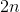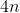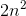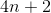Explanation:

For any n, the energy level can hold 2n2 electrons, since there are two electrons for each orbital.

### Example Question #1 : Quantum Numbers

What does the azimuthal quantum number reveal about the quantum mechanical model of an atom?

The orbital within the subshell that an electron is located in
The direction of spin of the electron
The number of valence electrons of the atom
The shape and number of subshells within the energy level
Correct answer: The shape and number of subshells within the energy level
Explanation:

The azimuthal quantum number is the second quantum number, designated by the letter l. It gives the shape and number of subshells in a principal energy level (shell).

### Example Question #1 : Quantum Numbers

Which of the following set of quantum numbers are not allowed: (a) n = 3, l = 2, ml = 0
(b) n = 2, l = 4, ml = –1 (c) n = 2.5, l = 1, ml = –1?

(a)

(c)

(b), (c)

(a), (b), (c)

(a), (b)

(b), (c)

Explanation:

The sets of quantum numbers needs to follow the following rules: n (principal quantum
number) needs to be a positive integer, l can have any integral value from 0 to n – 1, and ml can range from –l to l. The only quantum numbers that follows these rules are (a).

### Example Question #1 : Quantum Numbers

How does energy vary as the quantum number (n) of an orbital changes?

n is always constant, making the energy constant

As n increases, the energy increases as well

As n increases, energy decreases

n has no effect on energy

As n increases, the energy increases as well

Explanation:

The energy of an electron is related to the quantum number by the equation E = -R/n2, where R is constant. The negative charge make it so that as n increases, the numerical value of the energy becomes less negative, approaching zero. Thus the energy increases as n increases.

### Example Question #1 : Quantum Numbers

What is the angular momentum quantum number for the highest energy orbital in the ground state Manganese atom?

2

1

0

3

2

Explanation:

First, we will write out the electron configuration for the ground state Manganese atom, considering only the valence electrons: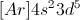The orbitals highest in energy will be filled last, so our highest energy orbitals are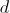orbitals.

The angular momentum quantum number describes the shape of the orbital, with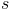orbitals corresponding to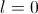,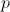orbitals corresponding with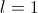, andorbitals corresponding to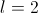, and so on.

We are considering aorbital, so.

### Example Question #2 : Quantum Numbers

Which of the following sets of quantum numbers could never be used to describe an electron?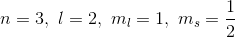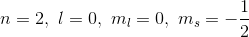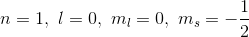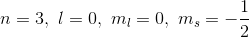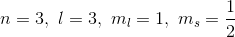Explanation:

The principle quantum number,, must always be an integer greater than zero.

The angular momentum quantum number,, can have values from zero up to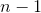.

The magnetic quantum number,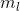, ranges from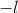to.

The spin quantum number,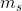, must beor.

To total, a set of quantum numbers follows the pattern: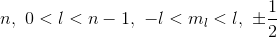The only answer option that presents an invalid set of quantum numbers is. In this option, the angular momentum quantum number,, has an illegal value; it must be between zero and, and thus cannot be equal to 3.

### Example Question #1 : Quantum Numbers

Which set of quantum numbers represents the highest energy valence electron in a ground-state aluminum atom?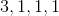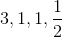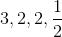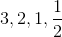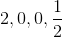Explanation:

The aluminum atom has its furthest electrons in the 3p shell. We know this because aluminum is in the third row of the periodic table, and is in the p-block. The possible quantum numbers for any valence electron are: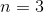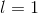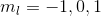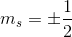### Example Question #8 : Quantum Numbers

Which of the quantum number describes the shape of the orbital where an electron is located?

ml

l

ms

n